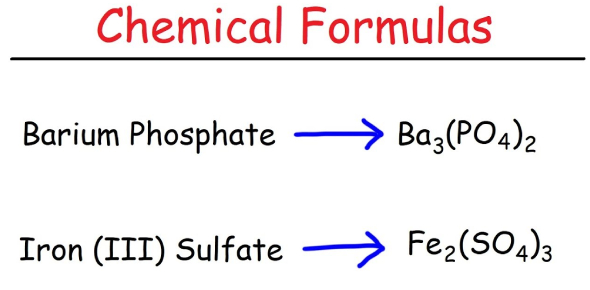# Year 10 Chemical Formulas Test

Approved & Edited by ProProfs Editorial Team
The editorial team at ProProfs Quizzes consists of a select group of subject experts, trivia writers, and quiz masters who have authored over 10,000 quizzes taken by more than 100 million users. This team includes our in-house seasoned quiz moderators and subject matter experts. Our editorial experts, spread across the world, are rigorously trained using our comprehensive guidelines to ensure that you receive the highest quality quizzes.
| By Antony Stubbs
A
Antony Stubbs
Community Contributor
Quizzes Created: 1 | Total Attempts: 10,610
Questions: 10 | Attempts: 10,627SettingsRevision of Chemical Formulas

This 10-item test will measure how well you understood the rules in writing down the correct chemical formulas of compounds. You have 5 minutes to complete the test. Identify the correct chemical formula of the given compound. Tick the letter that corresponds to your answer.

• 1.

### Calcium chloride

• A.
• B.

• C.
• D.

A.
Explanation
Calcium chloride is a chemical compound that is commonly used for a variety of purposes. It is often used as a desiccant to remove moisture from the air, as a food additive to provide a source of calcium, and as a de-icer to melt ice and snow on roads and sidewalks. Calcium chloride is also used in the oil and gas industry to increase the density of drilling fluids and to prevent the formation of clay and shale. Additionally, it is used in the production of cement, as a drying agent in industrial processes, and as a preservative for canned fruits and vegetables.

Rate this question:

• 2.

### Copper (II) oxide

C.
Explanation
Copper (II) oxide refers to the compound formed by the combination of copper and oxygen, where copper has a charge of +2. The Roman numeral II in the name indicates the oxidation state of copper in the compound. Copper (II) oxide is a black solid that is commonly used as a pigment and in the production of ceramics and glass. It can also act as a catalyst in various chemical reactions.

Rate this question:

• 3.

### Sodium hypochlorite

• A.

NaClO4

• B.

NaClO3

• C.

NaClO2

• D.

NaClO

D. NaClO
Explanation
Sodium hypochlorite is represented by the chemical formula NaClO. This compound is formed by the combination of sodium (Na) and hypochlorite (ClO). The correct answer is NaClO, as it matches the given chemical formula for sodium hypochlorite.

Rate this question:

• 4.

### Aluminum hydroxide

• A.

Al(OH)3

• B.

Al(OH)2

• C.

AlOH

• D.

AlO

A. Al(OH)3
Explanation
Aluminum hydroxide is a chemical compound with the formula Al(OH)3. The formula indicates that each aluminum atom is bonded to three hydroxide ions, which consist of one oxygen atom and one hydrogen atom. The correct answer, Al(OH)3, represents the correct formula for aluminum hydroxide, as it accurately reflects the composition and bonding of the compound. The other options, Al(OH)2, AlOH, and AlO, do not correctly represent the composition or bonding of aluminum hydroxide.

Rate this question:

• 5.

### Copper (II) sulfate

• A.

Cu2SO4

• B.

CuSO

• C.

CuSO4

• D.

Cu2(SO4)2

C. CuSO4
Explanation
The correct answer is CuSO4 because it represents the chemical formula for copper (II) sulfate. In this compound, copper has a charge of +2, indicated by the Roman numeral II in parentheses. Sulfate is a polyatomic ion with a charge of -2, represented by the formula SO4. Therefore, the correct formula for copper (II) sulfate is CuSO4.

Rate this question:

• 6.

### Potassium permanganate

• A.

KMnO5

• B.

KMnO4

• C.

KMnO3

• D.

KMnO2

B. KMnO4
Explanation
Potassium permanganate is represented by the chemical formula KMnO4. The correct answer, KMnO4, matches the given formula. The other options, KMnO5, KMnO3, and KMnO2, do not match the correct formula for potassium permanganate.

Rate this question:

• 7.

### Carbon dioxide

• A.

C2O

• B.

CO3

• C.

CO2

• D.

CO

C. CO2
Explanation
The correct answer is CO2 because carbon dioxide is a compound made up of one carbon atom and two oxygen atoms. The other options, C2O, CO3, and CO, do not have the correct combination of atoms to form carbon dioxide.

Rate this question:

• 8.

### Hydrochloric acid

• A.

HClO4

• B.

HClO

• C.

HClO3

• D.

HCl

D. HCl
Explanation
Hydrochloric acid is represented by the chemical formula HCl.

Rate this question:

• 9.

### Hyposulfurous acid

• A.

H2SO4

• B.

H2SO5

• C.

H2SO3

• D.

H2SO2

D. H2SO2
Explanation
H2SO2 is the correct answer because it corresponds to the compound hyposulfurous acid. The prefix "hypo-" indicates that there is one less oxygen atom compared to the common form of the acid. In this case, hyposulfurous acid has two hydrogen atoms and two oxygen atoms, represented by the formula H2SO2. The other options, H2SO4, H2SO5, and H2SO3, do not match the given compound.

Rate this question:

• 10.

### Ammonium acetate

• A.

NH4C2H3O2

• B.

NH4(C2H3O2)2

• C.

(NH4)2C2H3O2

• D.

(NH4)2(C2H3O2)2

A. NH4C2H3O2
Explanation
Ammonium acetate is a compound made up of the ammonium ion (NH4+) and the acetate ion (C2H3O2-). The formula NH4C2H3O2 represents one ammonium ion and one acetate ion combined together.

Rate this question:

Related TopicsBack to top